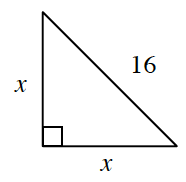### Home > GC > Chapter 5 > Lesson 5.3.5 > Problem5-115

5-115.Examine the triangle shown at right. Solve for $x$ twice, using two different methods. Show your work for each method clearly.

This triangle is similar to a $45º\text{-}45º\text{-}90º$ special right triangle.

A second way to solve for $x$ would be to use the Pythagorean Theorem.

Using the Pythagorean Theorem you get $x^2+x^2=16^2$
Combine like terms you get $2x^2=256$.
Solve for $x$ and simplify your radical.

$x=8\sqrt{2}$